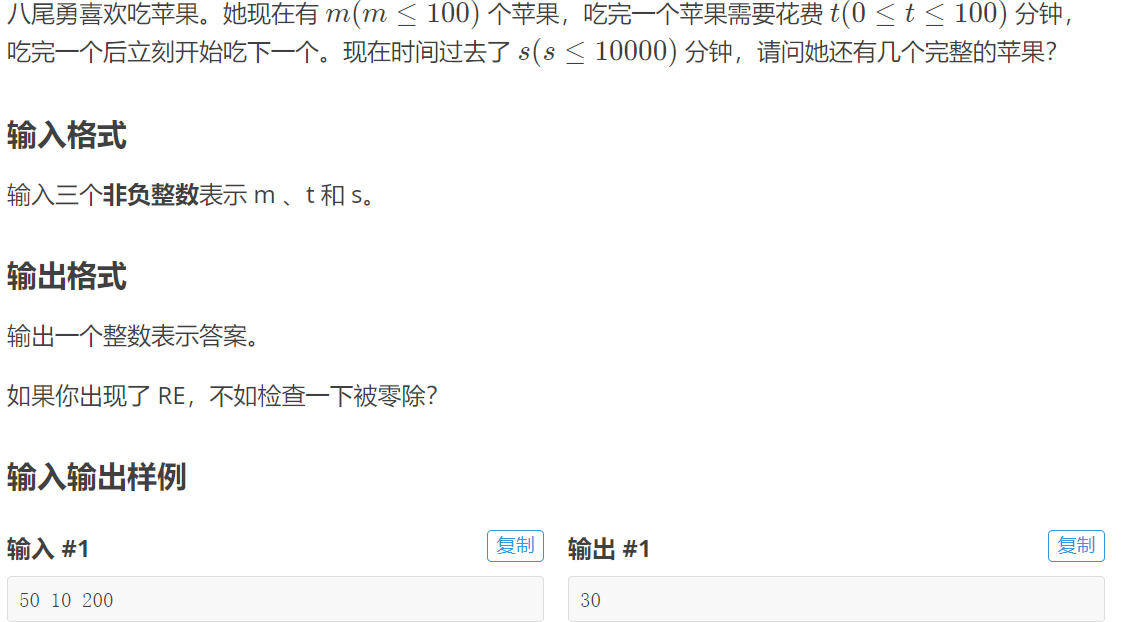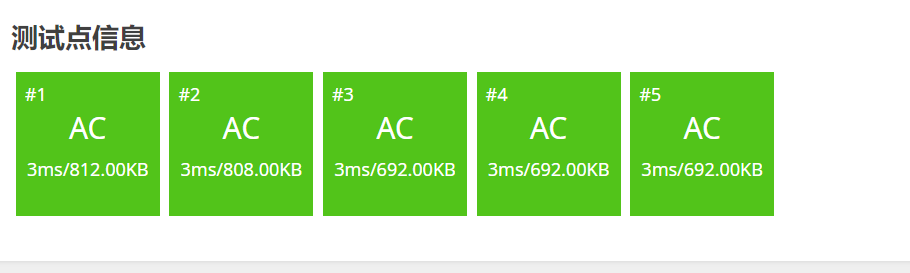• 洛谷-P5731 【深基5.习6】蛇形方阵
2021-05-22 03:21:04

洛谷-P5731 【深基5.习6】蛇形方阵

给出一个不大于 9 的正整数 $$n$$，输出 $$n\times n$$ 的蛇形方阵。

从左上角填上 1 开始，顺时针方向依次填入数字，如同样例所示。注意每个数字有都会占用 3 个字符，前面使用空格补齐。

输入 #1

4

输出 #1

1 2 3 4

12 13 14 5

11 16 15 6

10 9 8 7

#include

using namespace std;

int a;

int main() {

int n, x=1, y=0, k=1;

scanf("%d", &n);

while (k <= n*n) {

while (y

while (x

while (y>1 && !a[x][y-1]) a[x][--y]=k++;

while (x>1 && !a[x-1][y]) a[--x][y]=k++;

}

for (int i=1; i<=n; ++i) {

for (int j=1; j<=n; ++j)

printf("%3d", a[i][j]);

printf("\n");

}

return 0;

}

更多相关内容
• 代码如下： #include<stdio.h> int main() { int m,t,s; scanf("%d%d%d",&m,&t,&s); if(t==0) printf("0"); else { if(m-s/t>=0) { if(s%t==0) printf("%d",m-s/t);... re..代码如下：

#include<stdio.h>
int main()
{
int m,t,s;
scanf("%d%d%d",&m,&t,&s);
if(t==0)   printf("0");
else
{
if(m-s/t>=0)
{
if(s%t==0)   printf("%d",m-s/t);
else    printf("%d",m-s/t-1);
}
else   printf("0");
}
return 0;
}展开全文开发语言 后端
• 思路： 对有所的数进行遍历，如果可以被 num 整除，则执行 count1=count1+i ，则最终的结果就是可以被 num 整除的数之和，同样，如果不能整除，则执行 count2=count2+i，则最终的结果就是不能被 num 整除的数之和，...

## 思路：

对有所的数进行遍历，如果可以被 num 整除，则执行 count1=count1+i ，则最终的结果就是可以被 num 整除的数之和，同样，如果不能整除，则执行 count2=count2+i，则最终的结果就是不能被 num 整除的数之和，同时再除以两者的计数器 mark1、mark2。就可以得到最终结果。

## 代码：

#include<stdio.h>
#define MAX 10000
int main()
{
int All,num;
int j=0,k=0;
double count1=0,count2=0;
double mark1=0,mark2=0;
scanf("%d%d",&All,&num);
for(int i=1;i<All+1;i++)
{
if(i%num==0)
{
count1=count1+i;
mark1+=1;
}
else
{
count2=count2+i;
mark2+=1;
}
}
printf("%.1lf %.1lf",count1/mark1,count2/mark2);
}


## 小tips：

刚开始定义 count1 等数据用的是 float，结果有三个点通不过，下载测试点看了一下，发现就是小数点后面不一样，整数部分都差不多，应该是精确杜的问题吧，就把 float 换成了 double。然后就通过了。

展开全文• NOIP2005 普及组第一题 题目描述 陶陶家的院子里有一棵苹果树，每到秋天树上就会结出 1010 个苹果。苹果成熟的时候，陶陶就会跑去摘苹果。陶陶有个 3030 厘米高的板凳，当她不能直接用手摘到苹果的时候，就会踩到...

## 题目描述

陶陶家的院子里有一棵苹果树，每到秋天树上就会结出 1010 个苹果。苹果成熟的时候，陶陶就会跑去摘苹果。陶陶有个 3030 厘米高的板凳，当她不能直接用手摘到苹果的时候，就会踩到板凳上再试试。

现在已知 1010 个苹果到地面的高度，以及陶陶把手伸直的时候能够达到的最大高度，请帮陶陶算一下她能够摘到的苹果的数目。假设她碰到苹果，苹果就会掉下来。

## 输入格式

输入包括两行数据。第一行包含 1010 个 100100 到 200200 之间（包括 100100 和 200200 ）的整数（以厘米为单位）分别表示 1010 个苹果到地面的高度，两个相邻的整数之间用一个空格隔开。第二行只包括一个 100100 到 120120 之间（包含 100100 和 120120 ）的整数（以厘米为单位），表示陶陶把手伸直的时候能够达到的最大高度。

## 输出格式

输出包括一行，这一行只包含一个整数，表示陶陶能够摘到的苹果的数目。

## 输入输出样例

输入
100 200 150 140 129 134 167 198 200 111
110
输出
5

#include<stdio.h>
#define N 10
int main(void)

{   const int CHAIR = 30;
int a[N];
int max_height,num=0;
for (int i = 0; i < 10; ++i) {
scanf("%d",&a[i]);
}
scanf("%d",&max_height);
for (int i = 0; i < 10; ++i) {
if(a[i]<=max_height+CHAIR) num+=1;
}
printf("%d",num);
return 0;
}

展开全文• 思路： 这一题的思路很清晰啊，既然需要对每一个成员输入相关信息，且信息都是姓名，生日的年、月、日。那么定义一个结构体是在所难免的。同时因为要输入 1<n<100 个成员及相关信息，所以肯定不能一个一个实例...
• P1075 [NOIP2012 普及组] 质因数分解开发语言
• 洛谷P5737闰年展示 代码如下 #include <stdio.h> #include <stdlib.h> int year(int i) { return (i%4==0&&i%100!=0||i%400==0); } int main() { int x,y; scanf("%d %d",&x,&y); ...
•开发语言 后端
• ## 洛谷P5725 C语言题解

千次阅读 2021-11-14 11:41:01
#include<stdio.h> #include<math.h> #include<string.h> int main() { int i = 0; int j = 0; int n = 0; int a = 1; scanf("%d", &n); int arr; for (i = 0;... n...开发语言 后端
• 思路： 就硬算，丝毫不怂。定义一个二维数组 student ，放每个同学的编号和分数。再用三个 for 循环，对二维数组的每一层进行排序，再用两个 for 循环进行除最高分和最低分的平均分，放进数组 score 。...
• #include<stdio.h> int main() { int n,x; int m; //出现次数 scanf("%d%d",&n,&x); for(int i=1;i<=n;i++) { int b=i; while(b) //检索b中出现x的次数 { if(b%10==x) m++;......
• 代码如下 #include <stdio.h> #include <stdlib.h> int main() { int a,b,c,d; scanf("%c%c%c.%c",&a,&b,&c,&d); printf("%c.%c%c%c",d,c,b,a); return 0; }
• 很简单的思路： 写两个函数用来判断是否为偶数和是否大于4不大于12。在主函数里面调用这两个函数加以判断。 ———————————————————————————————— 代码如下： #include<...
• 该题很简单，定义一个数组，将10个苹果的高度全部输进去，再和陶陶能够到的最大高度作比较。 #include<stdio.h> int main() { int apple_high; for(int i=0;i<10;i++) ... for(...
• 啦啦啦坚持打代码一个赞敲一题？（qaq） #include<stdio.h> int main() { int i,n,count=1,max=0; long a={0}; scanf("%d",&n); for(i=0;i<n;i++) { scanf("%d",&... .
• #include <stdio.h> int main() { //采用倒序查找的方式，对点进行查找，如果没有找到对应的地毯，则输出-1。 //定义四个数组，以 i=0~num 的顺序将每层地毯的覆盖范围放进数组里，再 i=num~1 的顺序查找，...
•算法
• //P5731 【深基5.习6】蛇形方阵 //#define LOCAL #include <iostream> #include <cstdio> #include <cstring> #include <cmath> #include <algorithm> #define inf 0x3f3f3f3f #...
• #include<stdio.h> int main(){ int year; scanf("%d", &year); if( year < 1582){ return 0; } else{ if( (year % 4 == 0 && year %100 !=0) || (year % 400 == 0))... printf("1"...
• #include<stdio.h> int main(){ int num; scanf("%d", &num); if(num == 0){ printf("Today, I ate 0 apple."); } else if(num == 1){ printf("Today, I ate 1 apple.");... printf("...
• 代码如下： #include <stdio.h> int main(){ int n,line; scanf("%d",&n); for(int i=1;i<1000;i++){ if(n==1){ printf("1/1"); break; } if(n>i*(i+1)/2&&... ...
• 洛谷P1271就是这样的一个题目。事实就是，在昨晚之前，我从来没有去了解过快速排序的原理，在昨晚遇到这个题目，我查了一点资料，大概了解了一下快速排序的原理，它其实是对冒泡排序的一种优化，先从需要排序的...排序算法 算法
• 洛谷P2678]跳石头 ###Description这项比赛将在一条笔直的河道中进行,河道中分布着一些巨大岩石。组委会已经选择好了两块岩石作为比赛起点和终点。在起点和终点之间,有 N 块岩石(不含起点和终 点的岩石)。在比赛过程...
• #include<stdio.h> int main(){ int n, sum=0; scanf("%d", &n); for(int i=1; i<=n; i++){ sum += i; } printf("%d", sum); return 0; }
• 题目描述 求两数的积。 输入格式 两行，两个整数。 输出格式 一行一个整数表示乘积。 输入输出样例 输入 #1复制 1 2 输出 #1复制 2 说明/提示 每个数字不超过10^{2000}102000，需用高精。.....算法
• 题目描述幻方是一种很神奇的N*N矩阵：它由数字1,2,3,……,N*N构成，且每行、每列及两条对角线上的数字之和都相同。当N为奇数时，我们可以通过以下方法构建一个幻方：首先将1写在第一行的中间。之后，按如下方式从小...
• #include<stdio.h> #include<string.h> char a; int main(void) { int i; scanf("%s", a); for (i = 0; i < strlen(a); i++) if (a[i] >= 97 && a[i] <...}
• 题目传送门 作为月赛的第一题，还是蛮简单的。 思路 首先，不要被样例所迷惑，样例给出的只是一种可行的方案，但并不是最优解，最优解应是围出一个 2×22\times22×2 的像素格，所需的栅栏数为 (2+1)×2+(2+1)×2=12...c++
• 分数线划定 用冒泡排序过的，后来发现用快排更方便一点。 #include<stdio.h> int main() { int j,t,n,i,a,b,m,s; scanf("%d%d",&n,&m); for(i=1;i<...b[i],&...
• #include<stdio.h> #include<math.h> int sushu(int a)//判断一个数是否为质数 { int i=2; if(a==1) return 0; if(a==3||a==2) return 1; for(i=2;i<=sqrt(a);i++) { if(a%i==0) ... ......